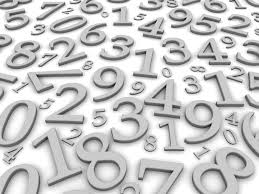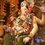# The Uninteresting Number!Every number is interesting. Take for example :

• $1$ is neither a prime nor composite
• $2$ is the first & the only even prime
• $3$ is the first odd prime
• $4$ is the first composite number

& so on.

But is there an uninteresting number? Let us find out.

Let $U$ be the set of all the uninteresting numbers.
Obviously, this set has a smallest element.

But such a number would be interesting because it is the first uninteresting number!

Hence, EVERY number is interesting!Note by Ameya Salankar
7 years, 1 month ago

This discussion board is a place to discuss our Daily Challenges and the math and science related to those challenges. Explanations are more than just a solution — they should explain the steps and thinking strategies that you used to obtain the solution. Comments should further the discussion of math and science.

When posting on Brilliant:

• Use the emojis to react to an explanation, whether you're congratulating a job well done , or just really confused .
• Ask specific questions about the challenge or the steps in somebody's explanation. Well-posed questions can add a lot to the discussion, but posting "I don't understand!" doesn't help anyone.
• Try to contribute something new to the discussion, whether it is an extension, generalization or other idea related to the challenge.
• Stay on topic — we're all here to learn more about math and science, not to hear about your favorite get-rich-quick scheme or current world events.

MarkdownAppears as
*italics* or _italics_ italics
**bold** or __bold__ bold
- bulleted- list
• bulleted
• list
1. numbered2. list
1. numbered
2. list
Note: you must add a full line of space before and after lists for them to show up correctly
paragraph 1paragraph 2

paragraph 1

paragraph 2

[example link](https://brilliant.org)example link
> This is a quote
This is a quote
    # I indented these lines
# 4 spaces, and now they show
# up as a code block.

print "hello world"
# I indented these lines
# 4 spaces, and now they show
# up as a code block.

print "hello world"
MathAppears as
Remember to wrap math in $$ ... $$ or $ ... $ to ensure proper formatting.
2 \times 3 $2 \times 3$
2^{34} $2^{34}$
a_{i-1} $a_{i-1}$
\frac{2}{3} $\frac{2}{3}$
\sqrt{2} $\sqrt{2}$
\sum_{i=1}^3 $\sum_{i=1}^3$
\sin \theta $\sin \theta$
\boxed{123} $\boxed{123}$

## Comments

Sort by:

Top Newest

Obviously, this set has a smallest element.

This is true if the word 'number' means a non-negative integer in this context. The well ordering principle works for non-negative integers only.

This seemingly pradoxical result arises because 'interesting' -ness is not a well-defined property. Would a number be considered interesting if it's uninteresting? Can something have a property $A$ by not having the property $A$?

- 7 years ago

Log in to reply

This is exactly how Agnishom Chattopadhyay won against me in a debate- " Are all people interesting" He was in the proposition and came up with something similar and said that being a bit uninteresting is in itself an interesting property...thus :P

- 7 years ago

Log in to reply

That was pretty interesting :)

Log in to reply

Nice proof!

- 7 years ago

Log in to reply

what about the 2nd unintresting number??????????? :P

- 7 years ago

Log in to reply

Its interesting for being the 2nd uninteresting number :D

- 7 years ago

Log in to reply

@Shreya R quite true!

- 7 years ago

Log in to reply

Using your method of contradiction, you have thrown away a seemingly extraneous solution.

It is more logically sound to say that all numbers are uninteresting, because in this case, a number isn't interesting, they are all uninteresting.

You've also stated

Obviously, this set has a smallest element

which is an assumption.

So really, no numbers are interesting.

- 7 years ago

Log in to reply

Obviously, this set has a smallest element

is not an assumption! It's common sense!

How come a set doesn't have a smallest element?

- 7 years ago

Log in to reply

Careful! A set doesn't have to have a smallest element. There is no smallest element in the set $S=\left\{ x : x \text{ is a country} \right\}$.

Even if you're talking about sets of numbers, the statement is not always true.

For example: $A=\left\{ x : x \in \mathbb{R}, 2 has no smallest element.

The well ordering principle works on non-negative integers only.

- 7 years ago

Log in to reply

OK! Looks like we have found a fallacy to the proof!

- 7 years ago

Log in to reply

That is not the fallacy actually. It is understood that the word 'number' means non-negative integer in this context. The real issue here is that 'interesting' is not well defined.

Here's a more interesting [pun intended!] variant of this.

Is it possible to describe every non-negative integer with $15$ words or less?

Here's an example. $4294967297$ is the first Fermat number that is not a prime. This description uses less than $15$ words. Is it possible to do that for every number?

Let's assume the contrary. Then set of numbers that can not be described with $15$ words or less is non-empty.

Now take the smallest element in this set. This is the smallest number that can not be described with $15$ words or less. Wait! That is a way to describe that number! Let's see how many words we used. I'll be damned! We used exactly $15$ words! This number does not belong in this set now. In a similar manner it can be shown that no number can be in this set.

The problem with this whole argument is that 'description' is not well defined. What counts as a 'description'? Is it okay for a description to be inconsistent?

- 7 years ago

Log in to reply

@Mursalin Habib, I knew this one. But there is something wrong with the proof. (The uninteresting number one)

- 7 years ago

Log in to reply

Yes,that's absolutely right.

- 7 years ago

Log in to reply

Yup...otherwise you're defying well ordering principle!!!

- 7 years ago

Log in to reply

What if it is an empty set? Then... :O

- 7 years ago

Log in to reply

@Finn Hulse, I forgot that one ... :D

- 7 years ago

Log in to reply

Hi-fi! We have the same number of followers! :D ^_^

- 7 years ago

Log in to reply

@Krishna Ar, you have caught up! Initially, you had less number of followers. Great job! Keep it up!

- 7 years ago

Log in to reply

Thank you ^_^. Initially I had way too less number of followers, yes now I have reached a decent level :D

- 7 years ago

Log in to reply

×

Problem Loading...

Note Loading...

Set Loading...